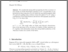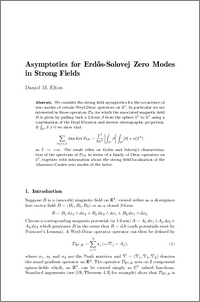# Asymptotics for Erdos-Solovej zero modes in strong fields

Elton, Daniel Mark (2016) Asymptotics for Erdos-Solovej zero modes in strong fields. Annales Henri Poincaré, 17 (10). pp. 2951-2973. ISSN 1424-0637Preview
PDF (ESzmasym)
ESzmasym.pdf - Accepted Version

## Abstract

We consider the strong field asymptotics for the occurrence of zero modes of certain Weyl-Dirac operators on $\R^3$. In particular we are interested in those operators $\Dirac{B}$ for which the associated magnetic field $B$ is given by pulling back a $2$-form $\beta$ from the sphere $\sphere$ to $\R^3$ using a combination of the Hopf fibration and inverse stereographic projection. If $\int_{\sphere}\beta\neq0$ we show that $\sum_{0\le t\le T}\dim\Ker\Dirac{tB} =\frac{T^2}{8\pi^2}\,\biggl\lvert\int_{\sphere}\beta\biggr\rvert\,\int_{\sphere}\abs{\beta}+o(T^2)$ as $T\to+\infty$. The result relies on Erd\H{o}s and Solovej's characterisation of the spectrum of $\Dirac{tB}$ in terms of a family of Dirac operators on $\sphere$, together with information about the strong field localisation of the Aharonov-Casher zero modes of the latter.

Item Type:
Journal Article
Journal or Publication Title:
Annales Henri Poincaré
The final publication is available at Springer via http://dx.doi.org/10.1007/s00023-016-0478-5
Uncontrolled Keywords:
/dk/atira/pure/subjectarea/asjc/3100/3106
Subjects:
Departments:
ID Code:
78739
Deposited By:
Deposited On:
16 Mar 2016 10:18
Refereed?:
Yes
Published?:
Published